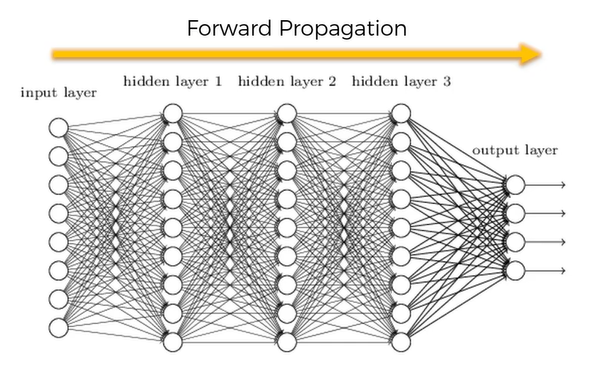# What is Artificial Neural Network(ANN)?

Original article was published by Manik Soni on Artificial Intelligence on Medium# What is Artificial Neural Network(ANN)?

## What is Artificial Neural Network(ANN)? How to do the Implementation of Artificial Neural Network(ANN)? Building a Deep Artificial Neural Network(ANN)

Prerequisites: Before we dive deep into Artificial Neural Network(ANN) we need to understand these 2 topics in order to understand what are neural networks.

# What is Artificial Neural Network(ANN)?

An Artificial Neural Network(ANN) is a computation system that is designed in a similar way to a human brain for doing the fast computation. It solves the problem that is statistically difficult.

# Steps to Do the Artificial Neural Network(ANN) with Stochastic Gradient Descent

Step 1. Randomly initialize the weights to a small number close to 0(but not 0).

Step 2. Input the first observation of your dataset in the input layer, each feature in one input node.

Step 3. Forward-Propagation: from left to right, the neurons are activated in a way that the impact of each neuron’s activation is limited by the weights. Propagate the activations until getting the predicted result y.

Step 4. Compare the predicted result to the actual result. Measure the generated error.

Step 5. Back-Propagation: from right to left, the error is backpropagated. Update the weights according to how much they are responsible for the error. The learning rate decides by how much we update the weights.

Step 6. Repeat Step 1 to 5 and update the weights after each observation(that is, Reinforcement Learning). Or Repeat Step 1 to 5 but update the weights only after a batch of observations(that is, Batch Learning).

Step 7. When the whole training set passed through the ANN, that makes an epoch( a particular slot). Redo more epochs.

# How to do the Implementation of Artificial Neural Network(ANN)?

In order to do the implementation of the Artificial Neural Network(ANN), we have to see the real-life application.

Dataset: We are importing the dataset of a bank account.

Problem: People are leaving the bank due to some reason, we need to find out the reason for the bank, what are there customers that are at higher risk of leaving the bank?

Building a Deep Artificial Neural Network(ANN)

Step 1. Importing the Libraries.

Step 2. Importing the dataset and categorize the dataset into a matrix of features(X) and dependent variable(y).

Step 3. Do the Label encoding to the categorical datasets.

Step 4. Do the feature Scaling in order to bring all the columns to the same scale.

Step 5. Splitting the dataset into training and test set.

Step 6. Initializing the ANN.

Step 7. Adding the 3 layers to ANN(input, output, and hidden layer )

Step 8. Now compiling the features for ANN and train our Neural Network.

Step 9. Now Predicting the Test set result.

Step 10. Making the confusion matrix to analyze the result.